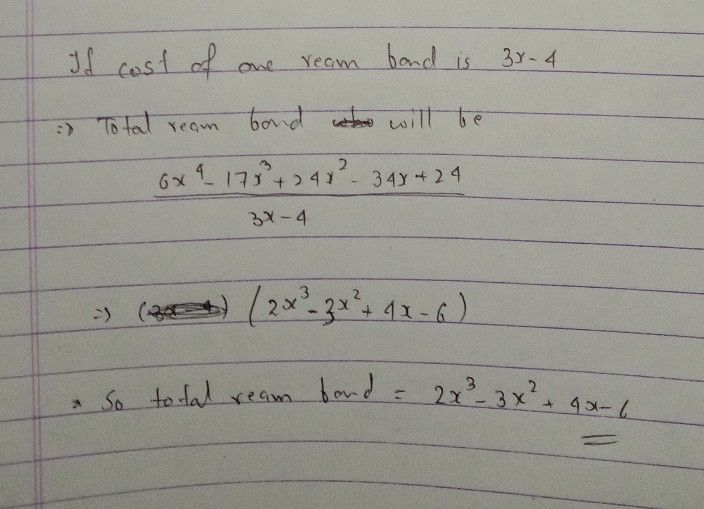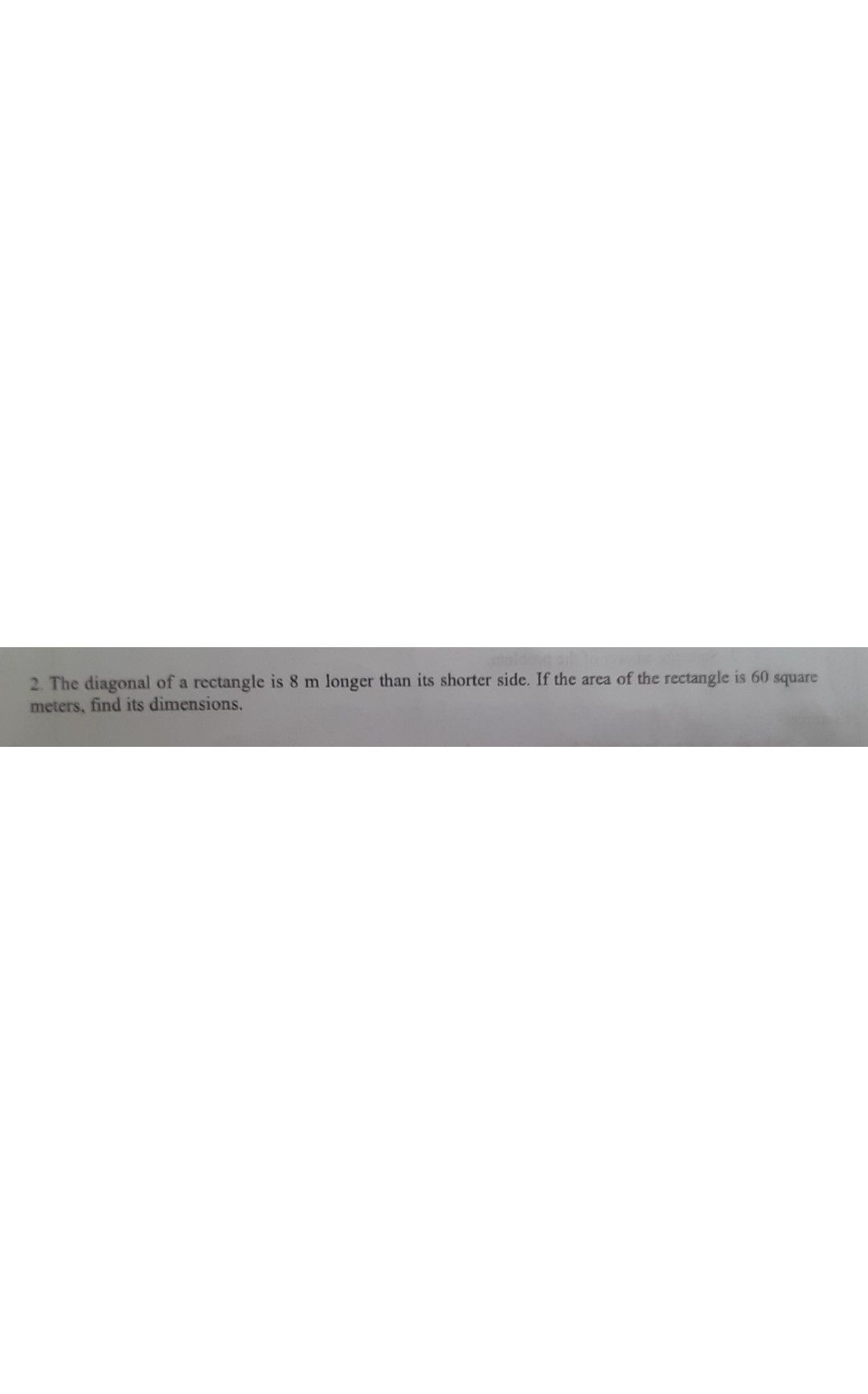Symbol
Problem$crc^{-\left(sc}$ Solve the following problems. $1$ If one ream of $oon$ paper costs $\left(3x-4\right)$ pesos, how many reams can you $bayf_{or\left(6x}4-17{x_{+24x}}^{32}-34x$ $+24\right)$ $2$ The diagonal of a rectangle is 8 m longer than its shorter side. If the area of the rectangle is 60 square meters, find its dimensions. $3.7$ The dimensions of a rectangular metal are $3cm$ $50$ 5cm and $8cm$ $l1$ the first two dimensions are increased by the same number of centimeters, while the third dimension remains the same, the new volume is 34cm' more than the original volume. What is the new dimension on the enlarged rectangular metal box $34c$ $4.0$ One dimension of a cube is increased by $1$ inch to form a rectangular block. Suppose that the volume of the new block is $150$ cubic inches, find the length of an edge of the original cube.
10th-13th grade
Other
Search count: 113
SolutionQanda teacher - AayushiStudent
thanks maamcan you answer this?